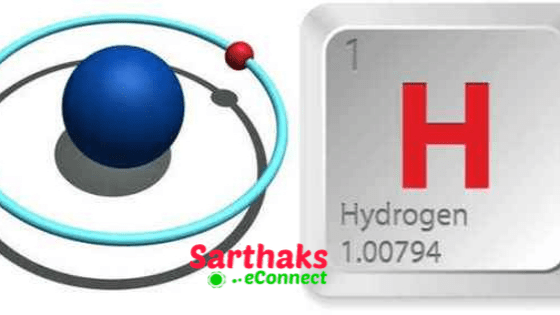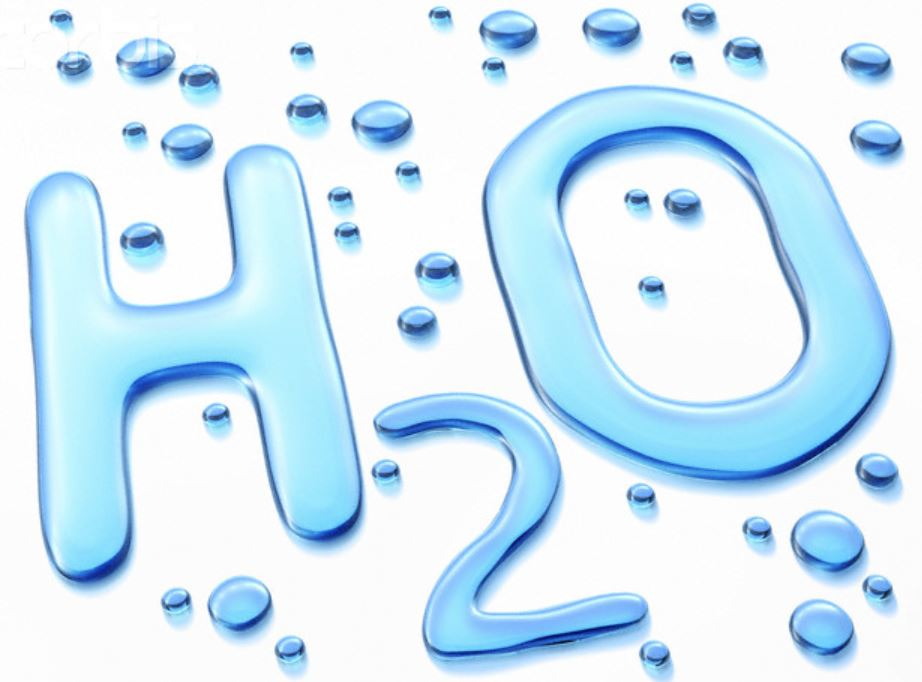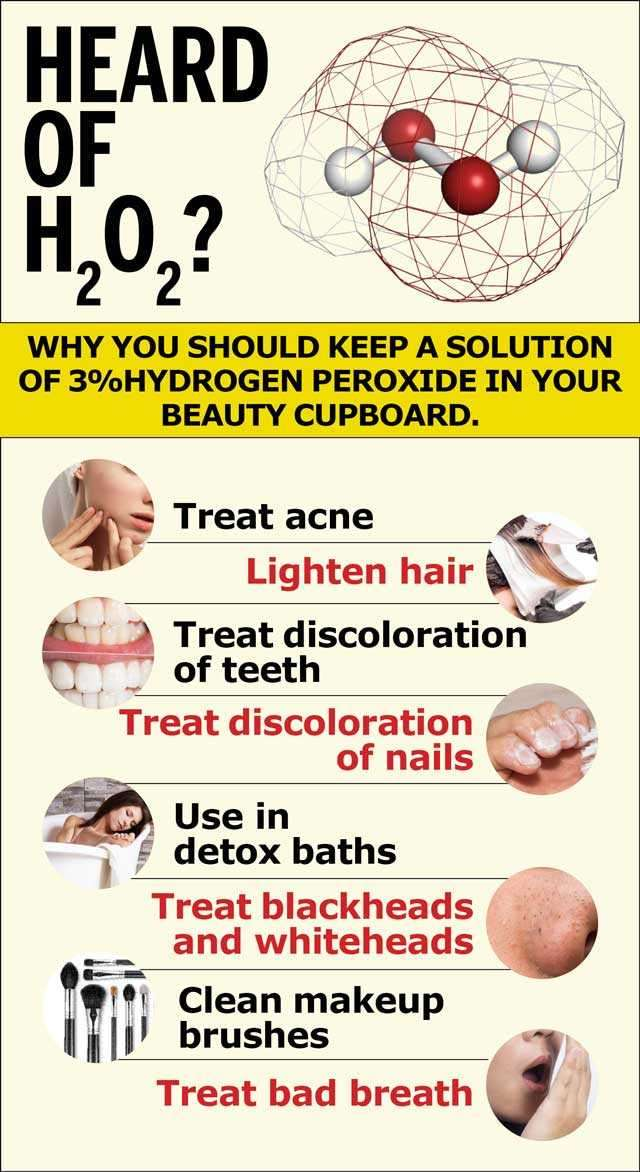## HydrogenHenry Cavendish discover hydrogen in 1766 by the action of dilute H2O4 on iron and named ‘inflammable air’, Lavoisier gave the name hydrogen (Creek: Hydra = water, gennas = producer]. These occurs in free state as well as in the combined state.

Lavoisier name hydrogen. Hydrogen is the 9th most abundant element in the earth’s crust.

Hydrogen exists in diatomic state but in the triatomic state it is Hyzone. Systematic name of water is oxidane.

### Position of Hydrogen in the Periodic Table:

Hydrogen resembles with alkali metals (group I) as well as halogens (group 17), At the same time, it differs from both in certain characteristics. That is why hydrogen if; called “rogue element”.

However. it has been placed in group 1 on the basis of its configuration 1s1, which is the basis of modern classification of elements.

• It forms monovalent H+ ion like Li+, Na+
•  valency is also 1.
• oxide (H2O) is stable as Li2O, Na2O.
• good reducing agent (In atomic as well as molecular state) like Na, Li…
• Hydrogen also resembles halogens (Group VII A)
• diatomic (H2)like F2, Cl2

#### Isotopes of Hydrogen

 Isotopes Relative atomic  mass Density Nature Protium $${H^1_1}$$ 1.0078 0.09 Non Radioactive Deuterium $${H^2_1}$$ 2.0141 0.18 Non Radioactive Tritium $${H^3_1}$$ 3.016 0.27 Radioactive

## Dihydrogen [H2]

Methods of Preparation:

### a) Lab methods

i) Zn + H2SO4 (aq) → ZnSO4 (aq) + H2 (g)

Metals which have reduction potential lesser than H, can liberate H2 from acids.

• Pure zinc is not used because it reacts slowly. The presence of some impurities increases the rate of reaction due to the formation of electrochemical couples.
• Conc sulphuric acid is also not used because it oxidises, H2 formed into H2O.

Zn + 2H2SO4 (conc.) → ZnSO4 + SO2 + 2H2O

ii) The reaction of zinc with aqueous alkali.

Zn + NaOH $$\overset{\Delta}{\rightarrow}$$ Na2ZnO2 + H2

#### b) Commercial production of dihydrogen

i) By the electrolysis of acidified water

 Electrode H2O ⇌ H+  +  OH– Ionisation At Cathode, H+  +  e– → H• Reduction H•  +  H• → H2 At Anode, 4OH– → 4OH  +  4e– Oxidation 4OH → 2H2O +  O2

ii) From water gas (Bosch process)

CO + H2 + H2O  $$\overset{Fe_2O_3 + Cr_2O_3}{\longrightarrow}$$ CO2  + O2

Carbon monoxide + hydrogen gas makes (Water-gas) +(Steam).Above reaction occurs in 773K

Carbon dioxide comes out by dissolving it in water under pressure (20-25 atm) and hydrogen left behind is obtain.

iii)  From steam (Lane’s process) Superheated steam is passed over iron filings heated to about 1023-1073 K when hydrogen is formed.

3Fe + 4H2O (Steam) $$\overset{1023 – 1073 K}{\rightarrow}$$ Fe3O4  + H2O

iv) Highly pure (> 99.95%)dihydrogen is obtained by electrolysing warm aqueous barium hydroxide solution between nickel electrodes.

v) From hydrocarbons by partial oxidation

CH4  + H2O $$\overset{Ni- Cr Catalyst}{\longrightarrow}$$ CO + 3H2

CH4  + H2O ( Natural gas + Steam). Above reaction occurs in (1270K)

vi) It is also obtained as a by-product in the manufacture of NaOH and chlorine by the electrolysis of brine solution.

During electrolysis, the reactions that take place are:

At Anode,  2Cl(aq) → Cl2(g) + 2e

At Cathode, 2H2O(l) +2e– →  H2(g) + 2OH(aq)

The overall reactions by separating Na+ ions,

2Na+(aq) + 2Cl(aq) + 2H2O(l) → Cl2(g) + H2(g)+ 2Na+ + 2OH (aq)

## Physical Properties of Dihydrogen

Dihydrogen is a colourless, odourless, tasteless, combustible gas. It is lighter than air and insoluble in water. It is neutral to litmus.

### Chemical Properties of Dihydrogen

i) Reactivity: The relative inertness of dihydrogen at room temperature is because of Its high enthalpy of H-H bond i.e.. high bond dissociation energy. So its reactions take place under specific conditions only (at high temperature).

ii) Action with non-metals:

2H2 + O2 $$\overset{970K}{\longrightarrow}$$ 2H2O(l); $$\Delta$$Ho = -285.9 kJ mol-1

N2 + 3H+ $$\overset{673K/200atm}{\longrightarrow}$$ 2NH3(g);$$\Delta$$Ho = -92.6kJ mol-1

H + X2 $$\overset{Dark}{\longrightarrow}$$ 2HX (X = halogens)

Order of reactivity of halogens:

F2 > Cl2 > Br2 > I2

iii) Reaction with metals Here H2 acts as oxidising agent.

2Na + H$$\overset{\Delta}{\rightarrow}$$ 2NaH

Ca + H $$\overset{\Delta}{\rightarrow}$$ CaH(Hydrolith)

iv) Reducing action of dihydrogen:

CuO + H$$\overset{\Delta}{\rightarrow}$$ Cu + H2O

v) Reactions with metal ions and metal oxides:

H2(g) + Pd+2(aq) → Pd(s) + 2H+(aq)

yH2(g) + MxOy(s) → xM(s) + yH2O(l)

vi) Reaction with organic compounds:

a)Veg.oil + H2  $$\overset{Ni/400K}{\longrightarrow}$$ Veg.ghee

b) R—CH = CH2 + H+CO  $$\overset{[Co(CO_4)_2]}{\longrightarrow}$$

RCH2CH2CHO + H $$\overset{Ni}{\longrightarrow}$$ RCH2CH2CH2OH

#### Uses of Dihydrogen

• In the manufacture of CH3OH.
• CO(g) + 2H(g)  $$\overset{Co}{\rightarrow}$$ CH3OH(l)
• It produces a temperature of 2850°C and oxy-atomic hydrogen flame produces a temperature of 4000°C, so it is used in oxy-hydrogen flame.
• The largest single use of H2 is in the synthesis of NH3 which is used in the manufacture ofHNO3 and fertilizers.
• We use liquid hydrogen (LH2) in fuel
• H2 is used as a reducing agent in extraction of metals.
• H2 is used in fuel cell for generating electrical energy.
• We is used in the manufacture of synthetic petrol.

(By heating H2 with coal and heavy oils under very high pressure in the presence of catalyst.)

## Different Forms of Hydrogen

### Atomic HydrogenH$$\overset{Ni/400K}{\rightarrow}$$ 2H $$\Delta$$Ho = 105.4 kcal mol-1

It is very reactive and its half-life period is 0.33 s.

#### Nascent Hydrogen

Freshly prepared hydrogen is known as nascent hydrogen and is more reactive than ordinary hydrogen. It causes the reduction of certain compounds which IS not possible with ordinary hydrogen. It can never be isolated.

Zn + H2SO4  → ZNSO4  +  2[H]

Adsorption of hydrogen at the metal surface is called occlusion. This hydrogen brings out many chemical changes such as reduction and hydrogenation. Occlusion decreases with rise in temperature.

###### Ortho and Para Hydrogen

When in hydrogen molecule, the nuclear spins are in the same direction, it is known as ortho hydrogen. On the other hand when the nuclear spins arc in the opposite direction. it is known as para hydrogen. At room temperature hydrogen consists of 75% ortho and 25% para hydrogen.

## Hydrogen EconomyThe hydrogen economy is the use of liquid hydrogen as an alternative source of energy. The technology involves the production, transportation and storage of energy in the form of liquid hydrogen. Large scale production of hydrogen can be done by electrolysis of water or by thermochemical reaction cycle. Storage of hydrogen in liquid form can be done in vacuum insulated cryogenic tanks or in a metal or in all alloy like iron-titanium alloy as an interstitial hydride. Hydrogen fuel has many advantage over conventional fuels in that it is non-polluting and it liberates a large amount of energy on combustion.

Photohydrogen is used to obtain renewable energy from sunlight by using microscopic organism such as bacteria or algae.

## Hydrides

The compounds of hydrogen with metals and non-metals are called hydrides.

### Ionic Hydrides

These are formed by elements of group I, II, (except Be and Mg) by heating them in hydrogen.

These are white colourless solids (crystalline) having high m.p. and b.p. easily decomposed by water, CO2 or SO2.

CaH2  +  2H2O → Ca  + (OH)2 + 2H2

CaH2 + 2CO2 → (HCOO)2Ca

They are strong reducing agents. Alkali metal hydrides are used for making LiAlH4, NaBH4, etc and for removing last traces of water from organic compounds.

#### Molecular or Covalent Hydrides

These are formed by elements of p-block having higher electronegativity than hydrogen.

Electron deficient hydrides: are the hydrides which do not have a sufficient number of electrons needed to form normal covalent bonds, e.g., BH3, AlH3, etc.
Precise: Which have exact number of electrons needed to form normal covalent bonds. e.g. hydrides of group 14 (CH4, SiH4, etc.)
Rich: They have greater number of electrons than require to form normal covalent bonds. e.g., hydrides of group 15, 16, 17, (NH3, PH3 ,H2S, HF, HCl, etc). The excess electrons in these hydrides are present as lone pairs of electrons.

##### Metallic or Interstitial Hydrides

The transition metals and rare earth metals combine with hydrogen to form interstitial hydrides. They exhibit metallic properties and are powerful reducing agents. They are non-stoichiometric hydrides and their composition varies with temperature and pressure for e.g., LaH2.76 TiH1.73

Metals of group 7, 8 and 9 do not form hydrides and this region of the Periodic Table is called hydride gap.

###### Polymeric Hydrides and Complex Hydrides

Polymeric hydrides are formed by’ elements having electronegativity in the range 1.4 to 2.0, e.g., (BeH2)n, (AlH3)n, etc. In complex hydrides, H– acts as ligand and is attached to the central metal atom, e.g., LiAlH4, LiBH4, etc

## WaterWater is the most abundant and widely distributed on the earth. It occurs in all three physical states. H2O is a covalent molecule in which oxygen is sp3 hybridised. It has a bent structure.

### Physical Properties of Water

• Water is a colourless, odourless, tasteless liquid. It has abnormally high b.p., f.p., the heat of vaporisation due to hydrogen bonding.
• Pure water is not a good conductor so it is made conductor by adding small amount of acid or alkali.
• The density of ice (which is mass per unit volume) is lesser than that of water and it floats over water.
• Water has a maximum density at 4°0.
• Water is a highly polar solvent with high dielectric constant 78.39. It interacts with polar or ionic substances effectively with the release of a considerable amount of energy due to ion-dipole interaction.
• The dissolution of covalent compounds like urea, glucose and C2H5OH, etc is due to the tendency of these molecules to form hydrogen bond with water.

#### Chemical Properties of Water

1) Water is amphoteric in nature.

H2O(l) + HCl(aq) ⇌  H3O+(aq) + Cl(aq)

H2O(l) + NH3(aq) ⇌ NH4+(aq) + OH(aq)

2) In redox reactions,water reacts with metals and non- metals both.

2Na(s) + 2H2O(l) → 2NaOH(aq) + H2(g)

3) In hydrated salts, water may remain in five types such as coordinated water, hydrogen bonded water, lattice water, clathrate water and zeolite water.

4) A number of compounds such as calcium hydride, calcium phosphide. etc ., undergo hydrolysis with water.

## Purification of Water

It involves two processes

a) Removal of suspended impurities
b) Destroying the bacteria.
Suspended particles are removed by coagulation with alum followed by filtration.

Exposure to sunlight, boiling, chlorination (treatment with liquid Cl2 or bleaching powder), ozonisation and addition of CuSO5 are some processes which are employed to destroy bacteria.

## Heavy Water [D2O]

It was discovered by Urey in 1932 and can be prepared by exhaustive electrolysis of ordinary water using nickel electrodes. They are colourless, odourless, tasteless liquid.

### Chemical Reactions of Heavy Water

 D2O reacts → Na SO3 CaC2 Al4C3 Ca3P2 Heavy Water NaOD +$$\frac{1}{2}$$ D2 D2SO4 Ca(OD)2 +C2D2 4Al(OD)3 + CD4 3Ca(OD)2 + 2PD3

#### Uses of Heavy Water1. In nuclear reactors to slow down the speed of neutrons and moderator.
2. As a tracer compound to study the mechanisms of many reactions.

## Soft and Hard Water

The water which produces a large amount of lather with soap is known as soft water and which forms scum with soap is known as hard water.

### Types of Hardness of Water in hydrogen

Temporary hardness: It is due to the presence of bicarbonates of calcium and magnesium.
Permanent hardness: It is due to the presence of chlorides and sulphates of calcium and magnesium.

#### Removal of Temporary Hardness

a) By boiling The soluble bicarbonates are converted into insoluble carbonates.

M(HCO3)2 → MCO3 ↓ + H2O + CO2 (M = Ca , Mg)

b) By Clark’s process By adding lime water or milk of lime.

M(HCO3)2 + Ca(OH)2 → MCO3 ↓ + 2H2O + CaCO3

##### Removal of Permanent Hardness

a) By adding washing soda The calcium or magnesium salts are precipitate as carbonates.

MgCl2 + Na2CO3 → MgCO3 ↓ + 2NaCl

b) By adding caustic soda The temporary and permanent hardness can be removed by adding caustic soda.

CaSO4  + 2NaOH →  Ca(OH)2 ↓ + Na2SO4

MgCl2 + 2NaOH →  Mg(OH)2 ↓ + 2NaCl

c) By adding sodium phosphate (Na3PO4) The phosphates of calcium and magnesium are precipitate.

3CaCl2 + 2Na3PO4 →  Ca3(PO4)2 ↓ + 6NaCl

3CaSO4 + 2Na3PO4 → Ca3(PO4)2 ↓ + 3Na2SO4

Similarly, magnesium also precipitate out in the form of magnesium phosphate, Mg3(PO4)2.

d) Calgon’s process Calgon is sodium hexa metaphosphate (Na6P6O18). This calgon when added to hard water form soluble complex.

2CaSO4 + Na2[Na4(PO3)6] → 2 Na2SO4 + Na2[Ca2(PO3)6]

Similarly. Mg2+ can also precipitate as Na2[Mg2(PO3)6] and water becomes free from Ca2+ and Mg2+ Ions.

e) Permutit process Permutit is hydrated sodium aluminium silicate Na2Al2Si2O8.xH2O. It exchanges its sodium ions for divalent ions Ca2+ and Mg2+

Na2Al2Si2O8 + MCl2 → MAl2Si2O8 + 2NaCl  (M = Ca , Mg)

Permutit when fully exhausted can be regenerated by treating with 10% solution of sodium chloride. It is most efficient method to gel water with zero degree of hardness.

###### By synthetic resins

These are of two types:

•  Cation exchange resins are big molecules containing sulphonic acid group (-SO3H). It is first changed into sodium salt with the general formula $$\overset{-}{R}$$ $$\overset{-}{Na}$$. The hard water is passed through it so Ca2+ and M2+ are exchanged and removed.

2RNa+ + Ca2+ → R2Ca + 2Na+

2RNa+ + Mg2+ → R2Mg + 2Na+

The resins like permutit can be regenerated with a solution of NaCl.

• Anion exchange resins are also big molecules and can exchange anions. They contain an amino group.

RNH2 + H2O → R$$\overset{+}{N}$$H3$$\overset{-}{O}$$H

The water is first passed through cation resins and then through anion resin and pure distilled water is form.

## Measurement of Degree of Hardness

Degree of hardness is the number of parts of calcium carbonate or equivalent to various calcium and magnesium salts present in one million parts of water by mass. It is expressed in ppm.

Degree of hardness (in ppm) = $$\frac{(wt. of CaCO_3(g))}{(wt. of hard water (g))}\times{10^6}$$

The molecular wt. of Ca(HCO3)2, Mg(HCO3)2, CaCl2, MgCl2, CaSO4 and MgSO4 is 162, 146, 111, 95, 136 and 120 respectively. The mol. wt. of CaCO3 is 100.

Thus, 162 g Ca(HCO3)2, 146 g Mg(HCO3)2, 111g CaCl2, 95g MgCl2 136g CaSO4 and 120 g MgSO4 are equivalent to 100 g CaCO3.

## Hydrogen Peroxide [H2O2]J.L. Thenard discover H2O2 in 1818. Hydrogen Peroxide used in pollution control treatment of domestic and industrial effluents.

### Methods of Preparation

i) BaO2 . 8H2O(s) + H2SO4(aq) → BaSO4(s) + H2O2(aq) + 8H2O(l)

ii) 2HSO4(aq) $$\overset{Electrolysis}{\longrightarrow}$$ HO3SOOSO3H(aq) $$\overset{H_2O}{\longrightarrow}$$ 2HSO4(aq) + 2H+(aq) + H2O2(aq)

iii) 2 −ethylanthraquinol $$\overset{O_2(air)}{\longrightarrow}$$ H2O2 + Oxidized product

#### Strength of Hydrogen PeroxideThe most common method to express the strength of H2O2 is in terms of the volume (in mL) of oxygen liberate at NTP by decomposition or 1 mL of that sample of H2O2.A solution of H2O2 take as ’10 volume’ actually means “1 mL of such a solution of H2O2 on decomposition by heat produces 10 mL of oxygen at NTP”.

##### Storage of Hydrogen Peroxide (H2O2)

It is store in the presence of traces of alcohol, acetanilide or sodium pyrophosphate which slow down the rate of decomposition of hydrogen peroxide.

###### Chemical Properties of H2O2

Acidic nature: It is weakly acidic in nature and pure hydrogen peroxide turns blue litmus red.

Oxidising agent: It acts as a strong oxidising agent in acidic as well as in basic medium.

Bleaching properties:Its bleaching action is due to oxidation by atomic oxygen and permanent.

H2O2 rarr; H2O + [O]

dye + [O] → dye is oxidised and bleached.

## Important Question & Answers in Hydrogen:

1.) Which pair does not show hydrogen isotopes

a) Ortho hydrogen and para hydrogen

b) Protium and deuterium

c) Deuterium and tritium

d) Tritium and protium

ANS:

Ortho and Para hydrogen show different spin in a hydrogen molecule it does not show hydrogen isotopes.

2.) On reaction with Mg, very dilute nitric acid produces:

a) NH3

b) Nitrous oxide

c) Nitric oxide

d) Hydrogen

ANS:

Mg + 2HNO3 → Mg(NO3)2 + H2

3.) Hydrogen resembles in many of its properties

a) Halogen

b) Alkali metals

c) Both a and b

d) None of these

4.) Hydrogen does not combine with:

a) Antimony

b) Sodium

c) Bismuth

d) Helium

ANS:

Helium is a noble gas and does not combine with hydrogen.

5.) Which of the following statements is most applicable to hydrogen

a) It can act as a reducing agent

b) It can act as an oxidising agent

c) It can act both as oxidising and reducing agent

d) It can neither act as oxidising nor as a reducing agent

ANS:

It acts both as a reducing agent and oxidising agent.